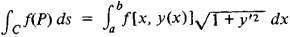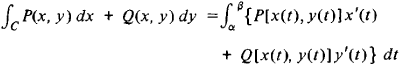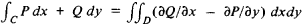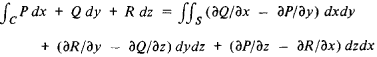Line Integral

Also found in: Wikipedia.

line integral

[′līn ¦int·ə·grəl]
(mathematics)
For a curve in a vector space defined byx=x(t), and a vector functionVdefined on this curve, the line integral ofValong the curve is the integral over t of the scalar product ofV[x(t)] and d x/ dt ; this is written ∫V· d x.
For a curve which is defined by x = x (t), y = y (t), and a scalar function ƒ depending on x and y, the line integral of ƒ along the curve is the integral over t of ƒ[x (t), y (t)] · √(dx / dt)2+ (dy / dt)2); this is written ∫ ƒ ds, where ds = √(dx)2+ (dy)2) is an infinitesimal element of length along the curve.
For a curve in the complex plane defined by z = z (t), and a function ƒ depending on z, the line integral of ƒ along the curve is the integral over t of ƒ[z (t)] (dz / dt); this is written ∫ ƒ dz.

Line Integral

an integral taken along some curve in the plane or in space. We distinguish line integrals of the first kind and line integrals of the second kind. A line integral of the first kind arises, for example, in problems involving the calculation of the mass of a curve of variable density and is denoted by

Cf (P)ds

where C is the given curve, ds is the differential of its arc, and f(P) is the function of a point on the curve and is the limit of the corresponding integral sums. In the case of a plane curve C given by the equation y = y(x), a line integral of the first kind reduces to an ordinary integral. Specifically,A line integral of the second kind arises, for example, in connection with the work of a force field. In the case of a plane curve C the integral takes the form

CP(x,y)dx + Q(x,y)dy

and is also the limit of the corresponding integral sums. A line integral of the second kind can be expressed as an ordinary integral. Specifically,wherex = x(t) and y = y(t) for α ≤ t ≤ β, is the parametric equation of the curve C. Its connection with a line integral of the first kind is given by the equality

CP(x,y)dx + Q (x,y)dy = ∫C {P cosα + Q sin α} ds

Here, α is the angle between the Ox axis and the tangent to the curve pointing in the direction of the increasing arc length.

A line integral of the second kind in space is defined similarly. (SeeVECTOR CALCULUS for a treatment of line integrals of the second kind from the standpoint of vectors.)

Suppose D is some region and C is its boundary. Under certain conditions, the line integral along the curve C and the double integral over the region D (see) are connected by the relationSimilarly, the line integral and the surface integral are connected by the relationLine integrals are of great importance in the theory of functions of a complex variable. They are widely used in various branches of mechanics, physics, and engineering.

References in periodicals archive ?
where Gauss's theorem over the horizontal plane is used, assuming no contributions from a far field line integral, and [(1 + [([eta]' -- [eta]).
3 The line integral of the field of a moving charge
Analytical expressions for reducing the highly oscillatory double PO integral to a line integral were given by Gordon [6, 7].
The most widely used scheme for producing pencil drawing effects is line integral convolution (LIC) , which integrates the noises scattered on the pixels of an image along an integration direction.
Complications due to the simultaneous nature of the price changes are handled with line integral theory.
If we restrict our attention far from the irregular boundary then the contribution of the branch line integral are small compared to the residue terms.
Each term of the series corresponds to a successive arrival of a "generalized ray" and each is a definite line integral along a fixed path which can be easily computed numerically.
More formally, instead of choosing a particular path of integration as in , write the correct welfare measure for a two-good price change in its general form as a line integral  [Mathematical Expression Omitted] where C is the path of integration between the prices ([Mathematical Expression Omitted]) and ([Mathematical Expression Omitted]) and [p.
i) The line integral [Mathematical Expression Omitted] taken along a closed contour in V is equal to zero.
From there, he says, it is possible to define properly the differential of a function, and from there, differential forms and pullbacks in the context of line integrals.
Chapters cover the complex plane, complex line integrals, applications of the Cauchy theory, Laurent series, the argument principle, the geometric theory, harmonic functions, infinite series and products, and analytic continuation.

Site: Follow: Share:
Open / Close